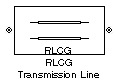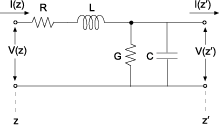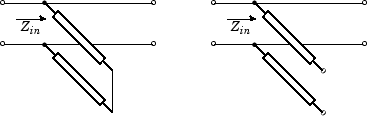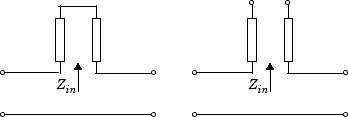# RLCG Transmission Line

Model RLCG transmission line

• Library:
• RF Blockset / Equivalent Baseband / Transmission Lines

•## Description

The RLCG Transmission Line block models the RLCG transmission line described in the block dialog box in terms of its frequency-dependent resistance, inductance, capacitance, and conductance. The transmission line, which can be lossy or lossless, is treated as a two-port linear network.where z′ = z + Δz.

To learn how you can use RLCG Transmission Line block to create baseband equivalent model, see Create Complex Baseband-Equivalent Model.

## Parameters

expand all

### Main

Resistance of the RLGC transmission lines, specified as a scalar in ohms/meters.

Inductance of the RLGC transmission lines, specified as a scalar in henries/meters.

Capacitance of the RLGC transmission lines, specified as a scalar in farad/meters

Conductance of the RLGC transmission lines, specified as a scalar in siemens per meter.

Vector of frequency values at which the resistance, inductance, capacitance, and conductance values are known.

Method to interpolate the network parameters, specified as one of the following:

MethodDescription
`Linear`Linear interpolation
`Spline`Cubic spline interpolation
`Cubic`Piecewise cubic Hermite interpolation

Physical length of the transmission line, specified as a scalar in meters.

The block enables you to model the transmission line as a stub or as a stubless line.

#### Stubless transmission Line

• `Not a stub`Not a stub

If you model a coaxial transmission line as stubless line, the Coaxial Transmission Line block first calculates the ABCD-parameters at each frequency contained in the modeling frequencies vector. It then uses the `abcd2s` function to convert the ABCD-parameters to S-parameters. For more information, see Stub Mode - Not a Stub.

#### Shunt Transmission Line

• `Shunt`—This parameter provides a two-port network that consists of a stub transmission line that you can terminate with either a short circuit or an open circuit as shown in these diagrams.Zin is the input impedance of the shunt circuit. The ABCD-parameters for the shunt stub are calculated as

`$\begin{array}{c}A=1\\ B=0\\ C=1/{Z}_{in}\\ D=1\end{array}$`

#### Series Transmission Line

• `Series`—This mode parameter provides a two-port network that consists of a series transmission line that you can terminate with either a short circuit or an open circuit as show in these diagrams.Zin is the input impedance of the series circuit. The ABCD-parameters for the series stub are calculated as

`$\begin{array}{c}A=1\\ B={Z}_{in}\\ C=0\\ D=1\end{array}$`

Stub termination for stub modes `Shunt` and `Series`. Choices are `Open` or `Short`

#### Dependencies

To enable this parameter, select `Shunt` or `Series` in Stub mode

### Visualization

Frequency data source, specified as `User-specified`.

Frequency data range, specified as a vector in hertz.

Reference impedance, specified as a nonnegative scalar in ohms.

Type of data plot to visualize using the given data, specified as one of the following:

• `X-Y plane` — Generate a Cartesian plot of the data versus frequency. To create linear, semilog, or log-log plots, set the Y-axis scale and X-axis scale accordingly.

• `Composite data` — Plot the composite data. For more information, see Create Plots.

• `Polar plane` — Generate a polar plot of the data. The block plots only the range of data corresponding to the specified frequencies.

• `Z smith chart`, ```Y smith chart```, and `ZY smith chart` — Generate a Smith® chart. The block plots only the range of data corresponding to the specified frequencies.

Type of parameters to plot, specified as one of the following.

 `S11` `S12` `S21` `S22` `GroupDelay` `GammaIn` `GammaOut` `VSWRIn` `VSWROut` `OIP3` `IIP3` `NF` `NFactor` `NTemp` `TF1` `TF2` `TF3` `Gt` `Ga` `Gp` `Gmag` `Gmsg` `GammaMS` `GammaML` `K` `Delta` `Mu` `MuPrime`

Note

Y parameter1 is disabled when you select Plot type to `Composite data`.

Type of parameters to plot, specified as one of the following.

 `S11` `S12` `S21` `S22` `GroupDelay` `GammaIn` `GammaOut` `VSWRIn` `VSWROut` `OIP3` `IIP3` `NF` `NFactor` `NTemp` `TF1` `TF2` `TF3` `Gt` `Ga` `Gp` `Gmag` `Gmsg` `GammaMS` `GammaML` `K` `Delta` `Mu` `MuPrime`

Note

Y parameter2 is disabled when you select Plot type to `Composite data`.

Plot format, specified as one of the following.

Y parameter1Y format1
`S11`, `S12`, `S21`, `S22`, `GammaIn`, `GammaOut`, `TF1`, `TF2`, `TF3`, `GammaMS`, `GammaML`, and `Delta`.`dB`, `Magnitude (decibels)`, `Abs`, `Mag`, `Magnitude (linear)`, `Angle`, `Angle(degrees)`, `Angle(radians)`, `Real`, `Imag`, and `Imaginary`.
`GroupDelay``ns`, `us`, `ms`, `s`, and `ps`.
`VSWRIn` and `VSWROut`.`Magnitude (decibels)` and `None`.
`OIP3` and `IIP3`.`dBm`, `W`, and `mW`.
`NF` `dB` and `Magnitude (decibels)`.
`NFactor`, `K`, `Mu`, and `MuPrime`.`None`
`NTemp``Kelvin`
`Gt`, `Ga`, `Gp`, `Gmag`, and `Gmsg`.`dB`, `Magnitude (decibels)`, and `None`.

#### Dependencies

To enable Y format1, set Plot type to `X-Y plane`.

Plot format, specified as one of the following.

Y parameter2Y format2
`S11`, `S12`, `S21`, `S22`, `GammaIn`, `GammaOut`, `TF1`, `TF2`, `TF3`, `GammaMS`, `GammaML`, and `Delta`.`dB`, `Magnitude (decibels)`, `Abs`, `Mag`, `Magnitude (linear)`, `Angle`, `Angle(degrees)`, `Angle(radians)`, `Real`, `Imag`, and `Imaginary`.
`GroupDelay``ns`, `us`, `ms`, `s`, and `ps`.
`VSWRIn` and `VSWROut`.`Magnitude (decibels)` and `None`.
`OIP3` and `IIP3`.`dBm`, `W`, and `mW`.
`NF` `dB` and `Magnitude (decibels)`.
`NFactor`, `K`, `Mu`, and `MuPrime`.`None`
`NTemp``Kelvin`
`Gt`, `Ga`, `Gp`, `Gmag`, and `Gmsg`.`dB`, `Magnitude (decibels)`, and `None`.

#### Dependencies

To enable Y format2, set Plot type to `X-Y plane`.

Frequency plot, specified as `Freq`.

Frequency plot format, specified as one of the following.

 `Auto` `Hz` `kHz` `MHz` `GHz` `THz`

Y-axis scale, specified as `Linear` or `Log`.

#### Dependencies

To enable this parameter, set Plot type to ```X-Y plane```.

X-axis scale, specified as `Linear` or `Log`.

#### Dependencies

To enable this parameter, set Plot type to ```X-Y plane```.

Plot the specified data using the plot button.